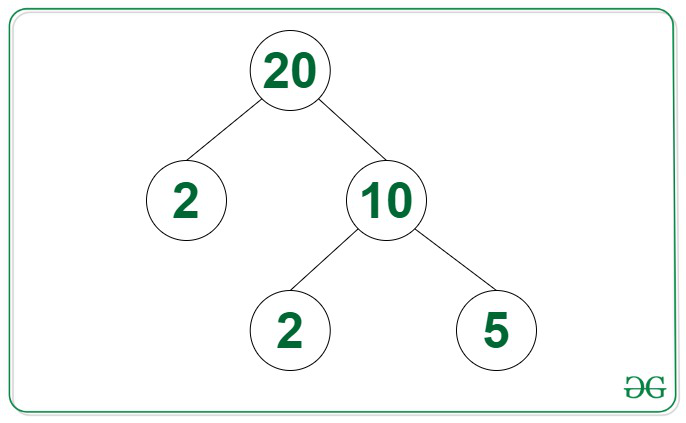# Height of Factor Tree for a given number

• Difficulty Level : Medium
• Last Updated : 08 Aug, 2022

Given a positive integer N, the task is to find the height of the Factor Tree of the given integer N.

Examples:

Input: N = 20
Output: 3
Explanation: The height of the Factor Tree of 20 shown in the image below is 3.Input: N = 48
Output: 5

Approach: The given problem can be solved by using the steps discussed below:

• Initialize a variable, say height as 0 that stores the height of the Factor Tree of the given integer N.
• Iterate over all the values of i in the range [2, √N] and perform the following steps:
1. Find the smallest divisor of N and if it exists, then increment the value of height by 1.
2. If a divisor i exists, then repeat the above step by updating the value of N to N / i, until N > 1.
3. If no divisors of N exist break the loop.
• After completing the above steps, print the value of height as the answer.

Below is the implementation of the above approach:

## C++

 `// C++ program for the above approach`   `#include ` `using` `namespace` `std;`   `// Function to find the height of the` `// Factor Tree of the integer N` `int` `factorTree(``int` `N)` `{` `    ``// Stores the height of Factor Tree` `    ``int` `height = 0;`   `    ``// Loop to iterate over values of N` `    ``while` `(N > 1) {` `        ``// Stores if there exist` `        ``// a factor of N or not` `        ``bool` `flag = ``false``;`   `        ``// Loop to find the smallest` `        ``// factor of N` `        ``for` `(``int` `i = 2; i <= ``sqrt``(N); i++) {` `            ``// If i is a factor of N` `            ``if` `(N % i == 0) {` `                ``N = N / i;` `                ``flag = ``true``;` `                ``break``;` `            ``}` `        ``}`   `        ``// Increment the height` `        ``height++;`   `        ``// If there are no factors of N` `        ``// i.e, N is prime, break loop` `        ``if` `(!flag) {` `            ``break``;` `        ``}` `    ``}`   `    ``// Return Answer` `    ``return` `height;` `}`   `// Driver Code` `int` `main()` `{` `    ``int` `N = 48;` `    ``cout << factorTree(N);`   `    ``return` `0;` `}`

## Java

 `// Java program for the above approach` `public` `class` `Main` `{` `    ``// Function to find the height of the` `    ``// Factor Tree of the integer N` `    ``static` `int` `factorTree(``int` `N)` `    ``{` `       `  `        ``// Stores the height of Factor Tree` `        ``int` `height = ``0``;` `      `  `        ``// Loop to iterate over values of N` `        ``while` `(N > ``1``)` `        ``{` `          `  `            ``// Stores if there exist` `            ``// a factor of N or not` `            ``boolean` `flag = ``false``;` `      `  `            ``// Loop to find the smallest` `            ``// factor of N` `            ``for` `(``int` `i = ``2``; i <= Math.sqrt(N); i++)` `            ``{` `              `  `                ``// If i is a factor of N` `                ``if` `(N % i == ``0``) {` `                    ``N = N / i;` `                    ``flag = ``true``;` `                    ``break``;` `                ``}` `            ``}` `      `  `            ``// Increment the height` `            ``height++;` `      `  `            ``// If there are no factors of N` `            ``// i.e, N is prime, break loop` `            ``if` `(!flag) {` `                ``break``;` `            ``}` `        ``}` `      `  `        ``// Return Answer` `        ``return` `height;` `    ``}` `    `  `    ``public` `static` `void` `main(String[] args) {` `        ``int` `N = ``48``;` `        ``System.out.println(factorTree(N));` `    ``}` `}`   `// This code is contributed by mukesh07.`

## Python3

 `# Python 3 program for the above approach` `from` `math ``import` `sqrt`   `# Function to find the height of the` `# Factor Tree of the integer N` `def` `factorTree(N):` `  `  `    ``# Stores the height of Factor Tree` `    ``height ``=` `0`   `    ``# Loop to iterate over values of N` `    ``while` `(N > ``1``):` `      `  `        ``# Stores if there exist` `        ``# a factor of N or not` `        ``flag ``=` `False`   `        ``# Loop to find the smallest` `        ``# factor of N` `        ``for` `i ``in` `range``(``2``,``int``(sqrt(N))``+``1``,``1``):` `            ``# If i is a factor of N` `            ``if` `(N ``%` `i ``=``=` `0``):` `                ``N ``=` `N ``/``/` `i` `                ``flag ``=` `True` `                ``break`   `        ``# Increment the height` `        ``height ``+``=` `1`   `        ``# If there are no factors of N` `        ``# i.e, N is prime, break loop` `        ``if` `(flag``=``=``False``):` `            ``break`   `    ``# Return Answer` `    ``return` `height`   `# Driver Code` `if` `__name__ ``=``=` `'__main__'``:` `    ``N ``=` `48` `    ``print``(factorTree(N))` `    `  `    ``# This code is contributed by SURENDRA_GANGWAR.`

## C#

 `// C# program for the above approach` `using` `System;` `class` `GFG {` `    `  `    ``// Function to find the height of the` `    ``// Factor Tree of the integer N` `    ``static` `int` `factorTree(``int` `N)` `    ``{` `      `  `        ``// Stores the height of Factor Tree` `        ``int` `height = 0;` `     `  `        ``// Loop to iterate over values of N` `        ``while` `(N > 1) {` `            ``// Stores if there exist` `            ``// a factor of N or not` `            ``bool` `flag = ``false``;` `     `  `            ``// Loop to find the smallest` `            ``// factor of N` `            ``for` `(``int` `i = 2; i <= Math.Sqrt(N); i++) {` `                ``// If i is a factor of N` `                ``if` `(N % i == 0) {` `                    ``N = N / i;` `                    ``flag = ``true``;` `                    ``break``;` `                ``}` `            ``}` `     `  `            ``// Increment the height` `            ``height++;` `     `  `            ``// If there are no factors of N` `            ``// i.e, N is prime, break loop` `            ``if` `(!flag) {` `                ``break``;` `            ``}` `        ``}` `     `  `        ``// Return Answer` `        ``return` `height;` `    ``}`   `  ``static` `void` `Main() {` `    ``int` `N = 48;` `    ``Console.Write(factorTree(N));` `  ``}` `}`   `// This code is contributed by decode2207.`

## Javascript

 ``

Output:

`5`

Time Complexity: O(√N*log N)
Auxiliary Space: O(1)

My Personal Notes arrow_drop_up
Recommended Articles
Page :